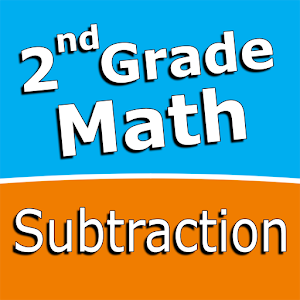# Second grade Math – SubtractionRating: 0/5

Intuitive interface powered by handwriting input and a fun and engaging mini game in addition to a regular math trainer mode make our app stand out from the crowd of generic math learning apps.

With Second grade Math – Subtraction you can practice and improve the following math skills:
– Subtraction facts – numbers up to 20
– Subtract a one-digit number from a two-digit number
– Subtract two two-digit numbers
– Subtract multiples of 10
– Subtract multiples of 100
– Subtract multiples of 10 or 100
– Subtract three-digit numbers
– Balance subtraction equations